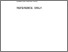# Power aspects of analysis of variance in various models.

KANJI, G. K. (1978). Power aspects of analysis of variance in various models. Doctoral, Sheffield Hallam University (United Kingdom)..Preview
PDF (Version of Record)
10697201.pdf - Accepted Version

## Abstract

The object of the present work is to study the robustness of the power in Analysis of Variance in relation to the departures from the in-built assumptions (i) equality of variance of the errors, (ii) statistical independence of the errors, and (iii) normality of the errors in fixed and random effects models. It is difficult if not impossible, to conduct an exhaustive study of the problem, because the above assumptions can be violated in many ways. However, a general model and some important particular models have been used to obtain fairly conclusive evidence regarding the robustness of the power in Analysis of Variance. In order to obtain the power value in relation to the departure from the usual test assumptions, the general linear hypothesis model is considered. The power values when the assumptions of equality of variances and independence of errors are violated, are obtained and presented in Table IA and IB. The result suggests that in the above model, for tests regarding the inference about means, the power value is greatly affected by the inequality of error variances but only slightly affected by the serially correlated error variables. By using the permutation theory an approximate method is developed to study the effect of non-normality of the errors on the probability of type two errors in the above situation. Having studied the most general case in Analysis of Variance some particular models are discussed to investigate certain important aspects of the problem that are generated by these models. First of all fixed model one-way classification is considered to investigate whether it could show a different picture for unequal replication. The results so obtained are presented in Table IIA and IIB. They indicate that the power value is greatly affected by the inequality of error variances and unequal group sizes. This procedure is easily modified to handle the random model. Another particular case of the general linear model, that is fixed effect model two-way classification, is discussed. The results so obtained are presented in Table IIIA and IIIB. They indicate that in two-way classification for the between Column test, the power value is greatly affected by the inequality of column variances but only slightly affected by the serially correlated within rows error variables. Again this procedure is easily modified to handle the random model. The use of simulation methods for calculating the power values in the case of non-normal errors is discussed. One and two-way classifications are considered for the fixed effect model. The Erlangian and contaminated normal distribution are taken as examples of a non-normal error distribution. The results obtained by these methods are given in Table IVA and IVB which indicate that for the inference concerning means, the power calculated under normal theory is only slightly affected by the non-normality of the errors. Finally, the effect of non-normality on the power in analysis of variance for a random effect model is also discussed by a simulation method. One and two-way classification are considered for this model and the Erlangian and contaminated normal distributions are taken as examples of non-normality. The results obtained by these methods are given in Tables VA and VB which indicate that non-normality has little effect on the power of the test.

Item Type: Thesis (Doctoral) Thesis (Ph.D.)--Sheffield Hallam University (United Kingdom), 1978. Sheffield Hallam Doctoral Theses EPrints Services 10 Apr 2018 17:20 26 Apr 2021 11:58 https://shura.shu.ac.uk/id/eprint/19895View Item